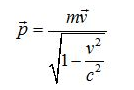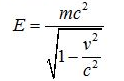# Problem: Relativistic Energy and MomentumLearning Goal:To learn to calculate energy and momentum for relativistic particles and, from the relativistic equations, to find relations between a particle's energy and its momentum through its mass.The relativistic momentum p and energy E of a particle with mass m moving with velocity v are given byandWhat is the rest mass m of a particle traveling with the speed of light in the laboratory frame.Express your answer in MeV/c2 to one decimal place.HintsHint 1. Equations for momentum and energyCompare the equations for energy and momentum if v=1.0c. What do you find when you plug these into E2−(pc)2 = m2c4?

###### FREE Expert Solution

The equivalence between mass and energy in the expression E2−(pc)= m2c4 gives the rest mass of a particle.
${\mathbit{E}}^{\mathbf{2}}\mathbf{=}\frac{{\mathbf{m}}^{\mathbf{2}}{\mathbf{c}}^{\mathbf{4}}}{\mathbf{1}\mathbf{-}\frac{{\mathbf{v}}^{\mathbf{2}}}{{\mathbf{c}}^{\mathbf{2}}}}$

${{\mathbf{\left(}}{\mathbit{p}}{\mathbit{c}}{\mathbf{\right)}}}^{{\mathbf{2}}}{\mathbf{=}}\frac{m2v2c2}{1-\frac{v2}{c2}}$

Taking the condition, v = 1.0c###### Problem Details

Relativistic Energy and Momentum

Learning Goal:

To learn to calculate energy and momentum for relativistic particles and, from the relativistic equations, to find relations between a particle's energy and its momentum through its mass.

The relativistic momentum p and energy E of a particle with mass m moving with velocity v are given byandWhat is the rest mass m of a particle traveling with the speed of light in the laboratory frame.

Hints

Hint 1. Equations for momentum and energy

Compare the equations for energy and momentum if v=1.0c. What do you find when you plug these into

E2−(pc)= m2c4?